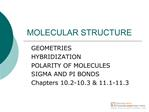DownloadDownload PresentationMOLECULAR STRUCTURE

MOLECULAR STRUCTURE

Télécharger la présentationMOLECULAR STRUCTURE

- - - - - - - - - - - - - - - - - - - - - - - - - - - E N D - - - - - - - - - - - - - - - - - - - - - - - - - - -
Presentation Transcript

Slide 1:MOLECULAR STRUCTURE

GEOMETRIES HYBRIDIZATION POLARITY OF MOLECULES SIGMA AND PI BONDS Chapters 10.2-10.3 & 11.1-11.3

Slide 2:Goals & Objectives

See the following Learning Objectives on pages 392 & 419. Understand these Concepts: 10.5-8 & 11.1-10. Master these Skills: 10.4-5 & 11.1-4.

Slide 3:MOLECULAR STRUCTURE

Draw the Lewis Electron Dot Structure Predict the electronic geometry Predict the hybridization on the central atom Predict the molecular geometry Predict the polarity of the molecule Predict the number of sigma bonds Predict the number of pi bonds

Slide 4:Electronic Geometry

VSEPR Theory Valence Shell Electron Pair Repulsion Theory -regions of high electron density about the central atom are as far apart as possible to minimize repulsions electronic geometries are determined by the number of regions of electron density about the central atom

Slide 5:Electronic Geometry

Regions of Electron Density Geometry 2 linear 3 trigonal planar 4 tetrahedral 5 trigonal bipyramidal 6 octahedral

Slide 6:VALENCE BOND THEORY

explains structures of molecules in terms of the overlap of atomic orbitals to produce bonds between atoms accounts for electronic geometries by a mathematical combination of atomic orbitals to produce hybrid orbitals that will fit the proposed electronic geometries

Slide 7:Hybrid Orbitals

Electronic Geometry Hybridization linear sp trigonal planar sp2 tetrahedral sp3 trigonal bipyramidal dsp3 octahedral d2sp3

Slide 8:sp Hybrid Orbitals

Figure 11.2 The sp hybrid orbitals in gaseous BeCl2. atomic orbitals hybrid orbitals orbital box diagrams Figure 11.2 The sp hybrid orbitals in gaseous BeCl2(continued). orbital box diagrams with orbital contours

Slide 11:sp2 Hybrid Orbitals

Figure 11.3 The sp2 hybrid orbitals in BF3.

Slide 13:sp3 Hybrid Orbitals

Figure 11.4 The sp3 hybrid orbitals in CH4. Figure 11.6 The sp3d hybrid orbitals in PCl5. Figure 11.7 The sp3d2 hybrid orbitals in SF6.

Slide 17:Molecular Geometry

Electronic Molecular Geometries Geometries linear linear trigonal planar trigonal planar angular tetrahedral tetrahedral angular trigonal pyramidal

Figure 10.7 The four molecular shapes of the trigonal bipyramidal electron-group arrangement. SF4 XeO2F2 IF4+ IO2F2- ClF3 BrF3 XeF2 I3- IF2- PF5 AsF5 SOF4 Figure 10.8 The three molecular shapes of the octahedral electron-group arrangement. SF6 IOF5 BrF5 TeF5- XeOF4 XeF4 ICl4-

Slide 20:Molecular Geometry

Figure 10.9 A summary of common molecular shapes with two to six electron groups.

Slide 22:Molecular Geometry

Slide 23:Molecular Geometry

Slide 24:Molecular Geometry

Slide 25:Molecular Geometry

Slide 26:POLARITY OF MOLECULES

the polarity of a molecule is the vector sum of the bond polarities of the molecule the bond polarities will either cancel or not

SAMPLE PROBLEM 10.9 Predicting the Polarity of Molecules (a) Ammonia, NH3 (b) Boron trifluoride, BF3 (c) Carbonyl sulfide, COS (atom sequence SCO) ENN = 3.0 ENH = 2.1 bond dipoles molecular dipole The dipoles reinforce each other, so the overall molecule is definitely polar. SAMPLE PROBLEM 10.10 Predicting the Polarity of Molecules continued (b) BF3 has 24 valence e- and all electrons around the B will be involved in bonds. The shape is AX3, trigonal planar. F (EN 4.0) is more electronegative than B (EN 2.0) and all of the dipoles will be directed from B to F. Because all are at the same angle and of the same magnitude, the molecule is nonpolar. 1200 (c) COS is linear. C and S have the same EN (2.0) but the C=O bond is quite polar(DEN) so the molecule is polar overall.

Slide 29:TYPES OF BONDS

SIGMA bonds head-on overlap of atomic orbitals all single bonds are sigma bonds PI bonds parallel overlap the extra bonds

Slide 30:TYPES OF BONDS

single bonds--sigma bonds double bonds--one sigma and one pi bond triple bonds-- one sigma and two pi bonds

Slide 31:CH4

Electronic geometry ____________ Hybridization on the central atom _______ Molecular geometry_____________ Polarity _________________ Number of sigma bonds ________ Number of pi bonds __________

Slide 33:CH4

Slide 34:C2H4

Electronic geometry _________________ Hybridization on the central atom _______ Molecular geometry _________________ Polarity _________________ Number of sigma bonds ________ Number of pi bonds __________

Slide 36:C2H4

Slide 37:C2H2

Electronic geometry _________________ Hybridization on the central atom _______ Molecular geometry _________________ Polarity _________________ Number of sigma bonds ________ Number of pi bonds __________

Slide 39:C2H2

Slide 40:CO32-

Electronic geometry _________________ Hybridization on the central atom _______ Ionic geometry _________________ Polarity _________________ Number of sigma bonds ________ Number of pi bonds __________

Slide 42:CO32-

Slide 43:BeI2

Electronic geometry _________________ Hybridization on the central atom _______ Molecular geometry _________________ Polarity _________________ Number of sigma bonds ________ Number of pi bonds __________

Slide 45:BCl3

Electronic geometry _________________ Hybridization on the central atom _______ Molecular geometry _________________ Polarity _________________ Number of sigma bonds ________ Number of pi bonds __________

Slide 47:NH3

Electronic geometry _________________ Hybridization on the central atom _______ Molecular geometry _________________ Polarity _________________ Number of sigma bonds ________ Number of pi bonds __________

Slide 49:H2O

Electronic geometry _________________ Hybridization on the central atom _______ Molecular geometry _________________ Polarity _________________ Number of sigma bonds ________ Number of pi bonds __________

Slide 51:SCN-

Electronic geometry _________________ Hybridization on the central atom _______ Ionic geometry _________________ Polarity _________________ Number of sigma bonds ________ Number of pi bonds __________

PCl5 Electronic geometry _________________ Hybridization on the central atom _______ Molecular geometry _________________ Polarity _________________ Number of sigma bonds ________ Number of pi bonds __________ SF6 Electronic geometry _________________ Hybridization on the central atom _______ Molecular geometry _________________ Polarity _________________ Number of sigma bonds ________ Number of pi bonds __________